0 Result
Your current location： Home > Mall News > Industry News > Quickly Create an Accurate Thermistor-Based Temperature Sensing Circuit
Hot Articles
Recommend Products

# Quickly Create an Accurate Thermistor-Based Temperature Sensing Circuit

2019-06-28Iczoom9

Temperature sensors are one of the most widely used sensors in the electronics industry with applications ranging from calibration; safety; and heating, ventilation, and air conditioning (HVAC). Despite their wide use, temperature sensors and their implementation can present designers with challenges in achieving the highest accuracy performance at the lowest possible cost.

There are a number of ways to sense temperature. The most common methods use temperature sensors such as the thermistor, resistance temperature detector (RTD), thermocouple, or a silicon thermometer. However, selecting the right sensor is only part of the solution. That sensor must then be connected to a signal chain that maintains the integrity of that signal, while also accurately compensating for the unique characteristics of the particular sensing technology to ensure an accurate digital representation of the temperature.

This article introduces a USB-powered circuit solution to accomplish this task. It uses a negative temperature coefficient (NTC) thermistor in combination with the Analog Devices ADuC7023BCPZ62I-R7 precision analog microcontroller to monitor temperature accurately.

NTC thermistor characteristics

A thermistor is a thermally sensitive resistor, of which there are two types: the positive temperature coefficient (PTC) thermistor and the NTC thermistor. The polycrystalline ceramic PTC thermistor has a high PTC and is typically used in switching applications. The NTC ceramic semiconductor thermistor has a high resistive NTC so its resistance decreases with increasing temperature. This makes it appropriate for precision temperature measurement.

There are three NTC thermistor modes of operation: resistance-versus-temperature, voltage-versus-current, and current-over-time. The mode that exploits the resistance-versus-temperature characteristics of the thermistor provides the highest precision results.

Resistance-versus-temperature circuits configure the thermistor in a “zero-power” condition. The “zero-power” condition assumes that current or voltage excitation of the device does not cause the thermistor to self-heat.

In a typical NTC thermistor, such as Murata Electronics’ NCP18XM472J03RB 4.7 kiloohm (kΩ) device in a 0603 package, the resistance-versus-temperature response is highly non-linear (Figure 1).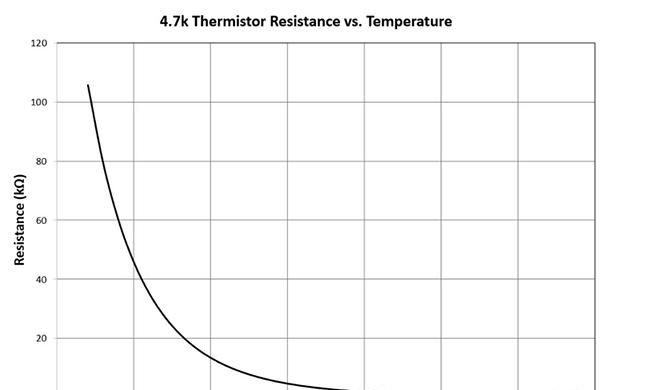Figure 1: The resistance-versus-temperature response of a typical NTC thermistor is highly non-linear, so designers need to find a way to bring this non-linearity under control for a defined temperature range. (Image source: Bonnie Baker, calculated and drawn based on resistance values from Murata)

The graph in Figure 1 shows the high degree of non-linearity from the 4.7 kΩ thermistor. The rate at which the resistance of an NTC thermistor decreases with temperature is a constant known as beta (ß) (not shown in Figure). For Murata’s 4.7 kΩ thermistor, β = 3500.

The correction of the thermistor’s non-linear response can be accomplished in software with a high-resolution analog-to-digital converter (ADC) and an empirical third-order polynomial or a look-up table.

However, there is a reasonable, easier, and less expensive hardware technique which, when applied before reaching the ADC, can bring the thermistor’s linearization problem under control for a ±25°C temperature range.

Hardware linearization solution

A simple approach to a first-level linearization of the thermistor output is to place the thermistor in series with a standard resistor (1%, metal film) and a voltage source. The value of the series resistor determines the median of the linear region of the thermistor circuit. The resistance value of the thermistor (RTH) and the Steinhart-Hart equation determine the thermistor’s temperature (Figure 2). The Steinhart-Hart equation has been found to be the best mathematical expression for determining an NTC thermistor’s temperature.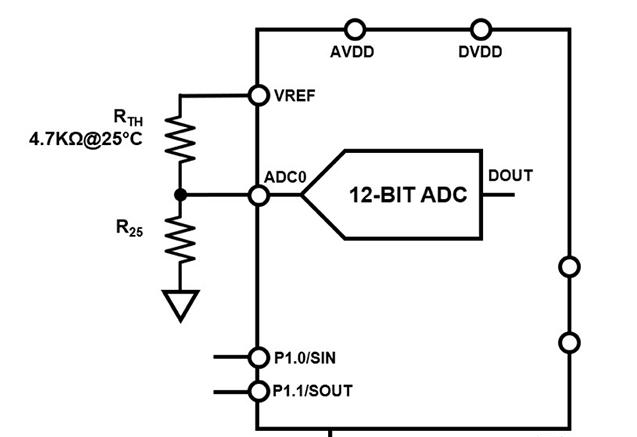Figure 2: A voltage divider (RTH and R25) configuration linearizes the thermistor’s response. The linear range at ADC0 (at the ADC input) is within a temperature range of approximately 50°C. (Image source: Bonnie Baker)

The derivation of the actual value of the thermistor’s resistance, RTH, starts by defining the voltage divider’s output (VADC0). VADC0 is then used to find the ADC’s digital output decimal code, DOUT, where DOUT depends upon the number of ADC bits (N), the ADC max input voltage (VREF), and the ADC input voltage (VADC0). The third and final step in finding RTH is to multiply R25 (or the 25°C RTH value) by the ratio of the number of ADC codes to the ADC’s digital output decimal code. This third step calculation process begins with Equation 2 below.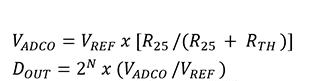The final step in the calculation is to convert the thermistor resistance to temperature in Kelvin units using the aforementioned Steinhart-Hart equation. The ADuC7023 precision analog microcontroller determines the sensor temperature using Equation 4: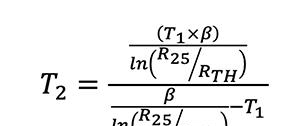Where:

T2 = Thermistor temperature being measured (in Kelvin units)

T1 = 298 Kelvin (25°C)

β = β parameter of the thermistor @ 298 Kelvin or 25°C. β = 3500

R25 = Thermistor resistance @ 298 Kelvin or 25°C. R25 = 4.7 kΩ

RTH = Resistance of thermistor @ unknown temperature, as calculated by Equation 3

In Figure 2, the thermistor resistance (RTH) is equal to 4.7 kΩ at 25°C. Since the value of R25 is equal to the thermistor’s 25°C value, the linear region of the voltage divider is centered around 25°C (Figure 3).Figure 3: Linear response of a 4.7 kΩ thermistor in series with a 4.7 kΩ standard resistor and 2.4 volts across the voltage divider. (Image source: Bonnie Baker, calculated and drawn based on resistance values from Murata)

In Figure 3, the series thermistor system responds to temperature linearly over a limited temperature range of approximately 0°C to +50°C. In this range, the delta temperature error is ±1°C. The linearization resistor’s value (R25) should be equal to the magnitude of the thermistor at the mid-point of the temperature range of interest.

This circuit acquires a 12-bit level of precision across a ±25°C temperature range typically, with the nominal temperature of the thermistor at the R25 value.

USB based temperature monitor

The signal path in the circuit solution starts with the low-cost, 4.7 kΩ thermistor followed by Analog Devices’ low-cost ADuC7023 microcontroller. The microcontroller integrates four 12-bit digital-to-analog converters (DACs), a multichannel 12-bit successive approximation register (SAR) ADC, and a 1.2 volt internal reference, as well as an ARM7® core, 126 Kbytes flash, 8 Kbytes static random access memory (SRAM), and various digital peripherals, such as a UART, timers, SPI, and two I2C interfaces (Figure 4).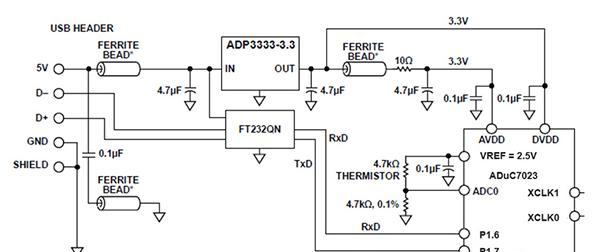Figure 4: The temperature sensing circuit uses a USB connection for power, and the ADuC7034 microcontroller’s I2C interface for digital communication. (Image source: Analog Devices)

In Figure 4, the power and ground to the circuit are entirely from the four-wire, USB interface. The Analog Devices ADP3333ARMZ-5-R7 low dropout linear regulator uses the USB 5 volt supply to generate a 3.3 volt output. The regulated ADP3333 output supplies the ADuC7023’s DVDD voltage. The ADuC7023’s AVDD supply requires additional filtering as shown. The linear regulator also has a filter between the USB supply and the IN pin.

The exchange of temperature data is also through the USB D+ and D- interface pins. The ADuC7023 is capable of using I2C protocol for transmitting and receiving data. This application circuit uses the two-wire I2C interface to transmit data and receive configuration commands.

This application uses the following ADuC7023 features:

An Arm ARM7TDMI with SRAM. An integrated 62 Kbytes of flash runs the user code that configures and controls the ADC, manages the communications over the USB interface and processes the ADC conversions from the thermistor sensor.

The I2C terminals are the communication interface to the host PC.

Two external switchesuttons (not shown) force the part into its flash boot mode: By holding DOWNLOAD low and toggling the RESET switch, instead of normal user mode the ADuC7023 enters the boot mode. The internal flash may be reprogrammed in the boot mode utilizing the USB interface through the device’s associated I2CWSD software tool.

VREF is the bandgap reference. This voltage reference is available for other circuit references in the system. A minimum of a 0.1 microfarad (μF) capacitor connects to these pins for noise reduction.

Since the ADuC7023 comes in a small form factor (5 mm × 5 mm) 32-pin chip scale package, the entire circuit fits on an extremely small section of a pc board, saving on cost and space.

The ADuC7023 provides a low-power solution even though it has a powerful ARM7 core and high-speed SAR ADC. The entire circuit typically consumes 11 milliamps (mA), with the ARM7 core running at 5 megahertz (MHz) and the primary ADC measuring the external thermistor. The microcontroller and/or the ADC can be switched off between temperature measurements, to further save on power consumption.

Layout considerations

The signal processing system shown in Figure 4 is surprisingly deceptive. From a bird’s eye view, this system only contains three active devices. But buried in this simplicity are some interesting layout challenges.

For example, the ADuC7023 microcontroller is a complex analog and digital system that requires special attention to the grounding rules. While this system may appear to be “slow moving” in the analog domain, its on-board track-and-hold ADC is a fast multi-channel device that samples at a rate of 1 mega sample per second, with a maximum clock speed of 41.78 MHz. In this system, the rise and fall times of the clock is a few nanoseconds. These speeds place this application in a high-speed category.

Clearly, mixed-signal circuits require special attention. Here’s a four point checklist encompassing the key aspects:

The use of electrolytic capacitors

The selection of the smaller capacitor

Ground plane considerations

A large electrolytic capacitor is typically used with a value of between 10 mF to 100 mF, located no more than two inches away from the chip. These capacitors act as charge reservoirs to accommodate the instantaneous charge requirement needs that come through power trace inductance.

The smaller capacitors in the circuit, typically ranging from 0.01 mF to 0.1 mF, are placed as physically close to the device’s power pins as possible. The purpose of these capacitors is to quickly and expeditiously send high frequency noise to ground.

The ground plane, below the decoupling capacitors, decouples high frequency currents and minimizes EMI/RFI emissions. It should consist of a large, low-impedance area. To minimize inductance, the capacitor connection to ground is through a via or a short trace.

In addition to Figure 4’s decoupling capacitors, EMI/RFI protection to the USB cable requires the use of ferrite. The ferrite beads in this circuit are Taiyo Yuden’s BK2125HS102-T which have an impedance of 1000 Ω at 100 MHz.

Conclusion

Temperature sensors are one of the most widely used sensors, yet design requirements continue to challenge designers to reduce cost and size, while improving sensing accuracy. Factoring in these considerations, this article described the implementation of a low-power, USB-based commercial thermistor system that uses a small 12-bit ADC and high-precision ADuC7023 microcontroller solution from Analog Devices. The combination successfully uses a resistor to tame an NTC thermistor with non-linear behavior to accurately sense and monitor temperature.

【Disclaimer】

1.The content, data, charts, etc. of this article come from network reference or other public materials, and the copyright belongs to the original author and the original published source. If the copyright owner has any objection to the quotation of this article, please contact ICZOOM "marketing(at)iczoom.com" and we will deal with it in a timely manner.

2.The quotes in this article are for readers' learning exchange only, and do not involve commercial purposes.

4.Please contact ICZOOM "marketing(at)iczoom.com" with the reason of reproducing if you want to reproduce the articles that ICZOOM owns the copyright. Without permission to reproduce, ICZOOM will reserve the right to pursue the legal liability.

5. If there is any inconsistency between the English and Chinese versions, the Chinese version shall prevail.

ICZOOM has the final right to interpret this statement.

keywords：Thermistor-Based,Temperature Sensing Circuit
Guideline
Return Instruction
Invoice
Disclaimer
Distribution
Distribution
Transportation Instruction
Tracking
Payment
E-bank
Bank Transfer
Alipay
Services
Loyalty Points